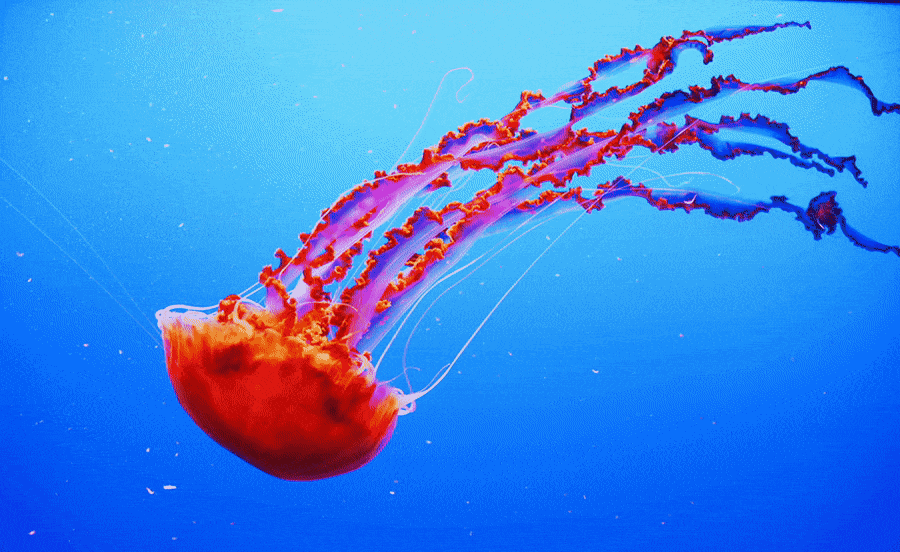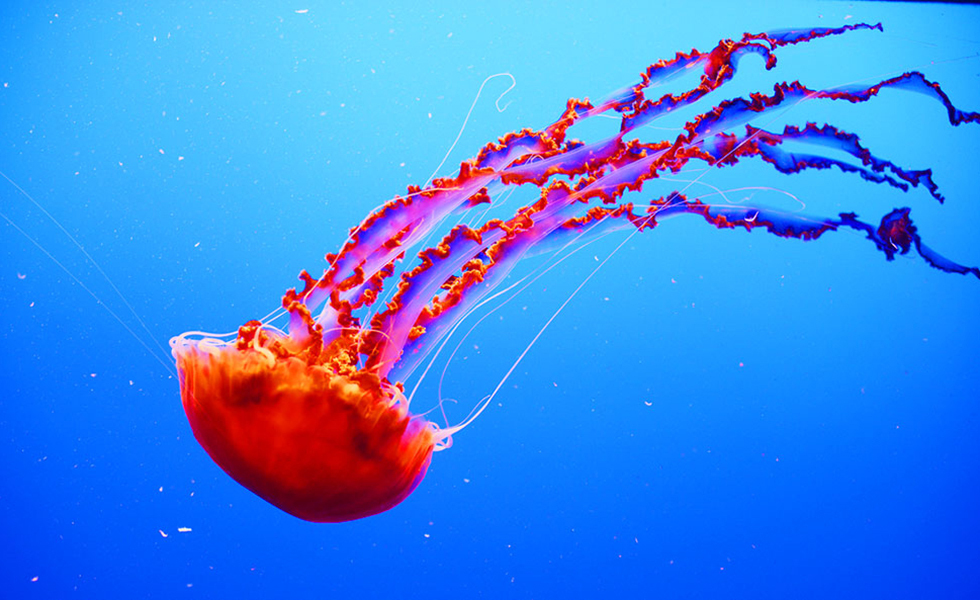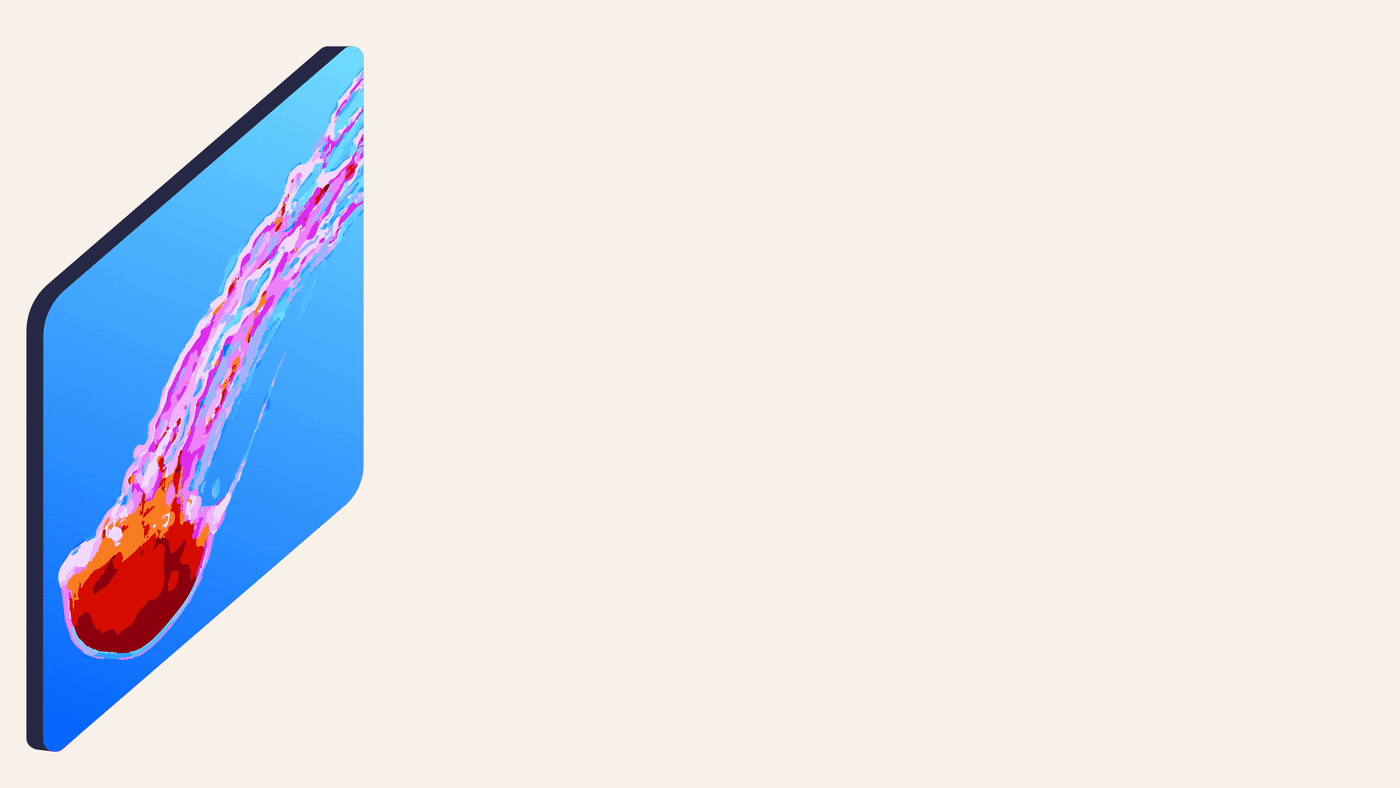# Delve into the layers of a deep learning model with GrAIdient

Duration:10 mins

Tags: AI / ML

Authors:

Date:March 6th, 2023

Share:

# Delve into the layers of a deep learning model with GrAIdientGrAIdient is Owkin’s newly-released open source framework for training and running deep learning models on Mac GPUs. In this article we will discuss two of its features: the direct access to the graph of layers that make up the neural network and to the backward pass (also known as backpropagation).

A matter of layers

Deep learning consists of machine learning models that are made up of a succession of layers. A layer is a structure within a deep learning neural network that carries information from previous layers to successive ones; this structure is present within all deep learning models. When running the model, successive layers create increasingly abstract representations of the input data. For example, when used with an image, the first layers may notice simple features like curves and edges, while later layers may start to recognize eyes, and even later layers, a face.

Popular deep learning frameworks, such as PyTorch, give flexibility to the user in how the model is structured. This flexibility allows the user to initialize layers’ weights in whatever order he or she prefers, but the user must specify the forward pass in a logical order. This introduces a discrepancy between the two orders and can lead to potentially redundant, incomplete and misleading information between the initialization and the real combination of these operations.

GrAIdient, meanwhile, has a “flat design” where the model is built as a graph of layers (a list where layers know their antecedent), that promotes clarity and consistency: the layers are initialized in the same logical order as the forward pass.

To visualize a model in this framework, let’s use a toy model you can find in the GrAIExamples directory. Originally, this toy model was used as an example to show that after some training, a small model is able to make better predictions. We’ll train this model to predict whether an image contains a jellyfish or not.In order to compute the model output on an image, such as the above jellyfish, we run each layer in the order of the forward pass. Here, we expect the model to output a 1, as the image is, in fact, a jellyfish. For an image that does not contain a jellyfish, we expect the model to output a 0.Initially, the model will often make incorrect predictions. During training, the model will progressively improve as it learns which features correlate to a correct prediction. Let us see how the learning process takes place.

The learning process

During the learning process, the framework gradually updates model parameters to improve prediction accuracy. These parameters are called weights and next, we’ll dive into the mechanisms used to update them.

First, GrAIdient computes the impact the current weights have had on the model’s final result and then it is able to update the weights so that the model’s result is closer to the expected output. The mathematical term for these impacts is gradients. They are computed during the backward pass which plays a prominent part in the learning process.

To provide confidence that the implementation of the backward function was done correctly, we use gradient checking, a technique that mathematically approximates each weights’ gradient in the model. For every weight, we operate as if the model contains only one weight to update: the specific weight for which we want to compute the gradient. Then, we compute two model outputs: one after having slightly increased the value of the weight, and another after having slightly decreased the value of the weight. By combining these two outputs, we are able to approximate the impact of the weight on the final output of the model.

One standard way of implementing this mechanism would be to run the original model twice as many times as the number of gradients we’re evaluating. This would generate numerous models to handle: 8762 in the case of our toy model! In GrAIdient, we prefer to work with one model, compute the approximations of the gradients from a layer perspective in a dedicated API, and call it forwardGC (short for "forward gradient checking"). With this design we have a better view of the 3 important flows that happen through the layers of our model: forward, backward and forwardGC.

Hence, with gradient checking natively implemented in GrAIdient, we are now able to create custom layers and demonstrate that the backward pass was correctly implemented. This mitigates the risk of user error thanks to the multiple unitary tests found in the GrAITests directory.

At Owkin, we think it is important to have a deep understanding of the mechanisms responsible for this learning process. The explicit definition of the backward function in every layer of GrAIdient gives the user an in-depth understanding of the model's inner mechanics. As we use deep learning on medical imaging data, as well as other patient data modalities, this deeper understanding is necessary for gathering information or biological insights from the data that may validate the model’s performance, and direct further study or inquiry. In the biomedical field, it is not enough to have a correct prediction for a datapoint; we always have to ask “why?”.

PyTorch interoperability

In addition to the custom layers which may be created in GrAIdient and the ones that are already implemented and ready for use, it is possible to reproduce other operations from PyTorch in GrAIdient. Due to interoperability between GrAIdient and PyTorch, we can create a model in GrAIdient, import the PyTorch model’s weights and run the whole model in GrAIdient to produce the same results as the PyTorch counterpart.

In the GrAITorchTests directory, tests run the same model in GrAIdient and PyTorch on one identical data input and reproduce the same gradients on the very first layer of the model. This ensures the different layers have produced the same output in the forward pass and then the same gradients in the backward pass; they run the exact same operation.

This use case is especially interesting for interpretability experiments at Owkin; we’re able to import a model that has been fully trained on PyTorch and conduct further experiments in GrAIdient. These experiments then fully benefit from the “flat design” of the GrAIdient model and the finer control over the gradients’ flow.

If you want to know more about GrAIdient mechanics visit the Github repository and connect with us.